For a linear regression model, a useful but underutilized diagnostic tool is the partial regression leverage plot. Also called the partial regression plot, this plot visualizes the parameter estimates table for the regression. For each effect in the model, you can visualize the following statistics:

• The estimate for each regression coefficient in the model.
• The hypothesis tests β0=0, β1=0, ..., where βi is the regression coefficient for the i_th effect in the model.
• Outliers and high-leverage points.

This article discusses partial regression plots, how to interpret them, and how to create them in SAS. If you are performing a regression that uses k effects and an intercept term, you will get k+1 partial regression plots.

### Example data for partial regression leverage plots

The following SAS DATA step uses Fisher's iris data. To make it easier to discuss the roles of various variables, the DATA step renames the variables. The variable Y is the response, and the explanatory variables are x1, x2, and x3. (Explanatory variables are also called regressors.)

In SAS, you can create a panel of partial regression plots automatically in PROC REG. Make sure to enable ODS GRAPHICS. Then you can use the PARTIAL option on the MODEL statement PROC REG statement to create the panel. To reduce the number of graphs that are produced, the following call to PROC REG uses the PLOTS(ONLY)=(PARTIAL) option to display only the partial regression leverage plots.

data Have; set sashelp.iris(rename=(PetalLength = Y PetalWidth = x1 SepalLength = x2 SepalWidth = x3) where=(Species="Versicolor")); ID = _N_; label Y= x1= x2= x3=; run;   ods graphics on; title "Basic Partial Regression Leverage Plots"; proc reg data=Have plots(only)=(PartialPlot); model Y = x1 x2 x3 / clb partial; ods select ParameterEstimates PartialPlot; quit;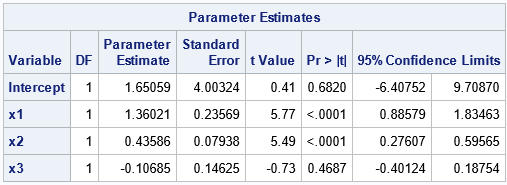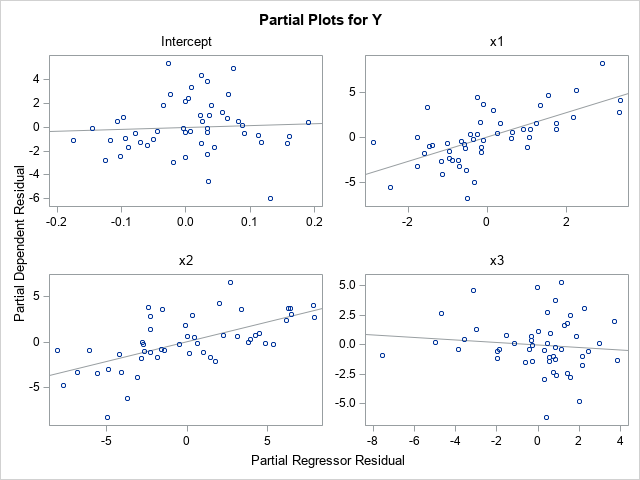Let's call the parameter for the Intercept term the 0th coefficient, the parameter for x1 the 1st coefficient, and so on. Accordingly, we'll call the upper left plot the 0th plot, the upper right plot the 1st plot, and so on.

A partial regression leverage plot is a scatter plot that shows the residuals for a specific regressions model. In the i_th plot (i=0,1,2,3), the vertical axis plots the residuals for the regression model where Y is regressed onto the explanatory variables but omits the i_th variable. The horizontal axis plots the residuals for the regression model where the i_th variable is regressed onto the other explanatory variables. For example:

• The scatter plot with the "Intercept" title is the 0th plot. The vertical axis plots the residual values for the model that regresses Y onto the no-intercept model with regressors x1, x2, and x3. The horizontal axis plots the residual values for the model that regresses the Intercept column in the design matrix onto the regressors x1, x2, and x3. Thus, the regressors in this plot omit the Intercept variable.
• The scatter plot with the "x1" title is the 1st plot. The vertical axis plots the residual values for the model that regresses Y onto the model with regressors Intercept, x2, and x3. The horizontal axis plots the residual values for the model that regresses x1 onto the regressors Intercept, x2, and x3. Thus, the regressors in this plot omit the x1 variable.

These plots are called "partial" regression plots because each plot is based on a regression model that contains only part of the full set of regressors. The i_th plot omits the i_th variable from the set of regressors.

### Interpretation of a partial regression leverage plot

Each partial regression plot includes a regression line. It is this line that makes the plot useful for visualizing the parameter estimates. The line passes through the point (0, 0) in each plot. The slope of the regression line in the i_th plot is the parameter estimate for the i_th regression coefficient (βi) in the full model. If the regression line is close to being horizontal, that is evidence for the null hypothesis βi=0.

To demonstrate these facts, look at the partial regression plot for the Intercept. The partial plot has a regression line that is very flat (almost horizontal). This is because the parameter estimate for the Intercept is 1.65 with a standard error of 4. The 95% confidence interval is [-6.4, 9.7]. Notice that this interval contains 0, which means that the flatness of the regression line in the partial regression plot supports "accepting" (failing to reject) the null hypothesis that the Intercept parameter is 0.

In a similar way, look at the partial regression plot for the x1 variable. The partial plot has a regression line that is not flat. This is because the parameter estimate for x1 is 1.36 with a standard error of 0.24. The 95% confidence interval is [0.89, 1.83], which does not contain 0. Consequently, the steepness of the slope of the regression line in the partial regression plot visualizes the fact that we would reject the null hypothesis that the x1 coefficient is 0.

The partial regression plots for the x2 and x3 variables are similar. The regression line in the x2 plot has a steep slope, so the confidence interval for the x2 parameter does not contain 0. The regression line for the x3 variable has a negative slope because the parameter estimate for x3 is negative. The line is very flat, which indicates that the confidence interval for the x3 parameter contains 0.

John Sall ("Leverage Plots for General Linear Hypotheses", TAS, 1990) showed that you could add a confidence band to the partial regression plot such that if the line segment for Y=0 is not completely inside the band, then you reject the null hypothesis that the regression coefficient is 0.

### Identify outliers by using partial regression leverage plots

Here is a remarkable mathematical fact about the regression line in a partial regression plot: the residual for each observation in the scatter plot is identical to the residual for the same observation in the full regression model! Think about what this means. The full regression model in this example is a set of 50 points in four-dimensional space. The regression surface is a 3-D hyperplane over the {x1, x2, x3} variables. Each observation has a residual, which is obtained by subtracting the predicted value from the observed value of Y. The remarkable fact is that in each partial regression plot, the residuals between the regression lines and the 2-D scatter points are exactly the same as the residuals in the full regression model. Amazing!

One implication of this fact is that you can identify points where the residual value is very small or very large. The small residuals indicate that the model fits these points well; the large residuals are outliers for the model.

Let's identify some outliers for the full model and then locate those observations in each of the partial regression plots. If you run the full regression model and analyze the residual values, you can determine that the observations that have the largest (magnitude of) residuals are ID=15, ID=39, ID=41, and ID=44. Furthermore, the next section will look at high-leverage points, which are ID=2 and ID=3. Unfortunately, the PLOTS=PARTIALPLOT option does not support the LABEL suboption, so we need to output the partial regression data and create the plots manually. The following DATA step adds a label variable to the data and reruns the regression model. The PARTIALDATA option on the MODEL statement creates an ODS table (PartialPlotData) that you can write to a SAS data set by using the ODS OUTPUT statement. That data set contains the coordinates that you need to create all of the partial regression plots manually:

/* add indicator variable for outliers and high-leverage points */ data Have2; set Have; if ID in (2,3) then Special="Leverage"; else if ID in (15,39,41,44) then Special="Outlier "; else Special = "None "; if Special="None" then Label=.; else Label=ID; run;   proc reg data=Have2 plots(only)=PartialPlot; model Y = x1 x2 x3 / clb partial partialdata; ID ID Special Label; ods exclude PartialPlotData; ods output PartialPlotData=PD; quit;

You can use the PD data set to create the partial regression plot. The variables for the horizontal axis have names such as Part_Intercept, Part_x1, and so forth. The variables for the vertical axis have names such as Part_Y_Intercept, Part_Y_x1, and so forth. Therefore, it is easy to write a macro that creates the partial regression plot for any variable.

%macro MakePartialPlot(VarName); title "Partial Leverage Plot for &VarName"; proc sgplot data=PD ; styleattrs datasymbols=(CircleFilled X Plus); refline 0 / axis=y; scatter y=part_Y_&VarName x=part_&VarName / datalabel=Label group=Special; reg y=part_Y_&VarName x=part_&VarName / nomarkers; run; %mend;

The following ODS GRAPHICS statement uses the PUSH and POP options to temporarily set the ATTRPRIORITY option to NONE so that the labeled points appear in different colors and symbols. The program then creates all four partial regression plots and restores the default options:

ods graphics / PUSH AttrPriority=None width=360px height=270px; %MakePartialPlot(Intercept); %MakePartialPlot(x1); %MakePartialPlot(x2); %MakePartialPlot(x3); ods graphics / POP; /* restore ODS GRAPHICS options */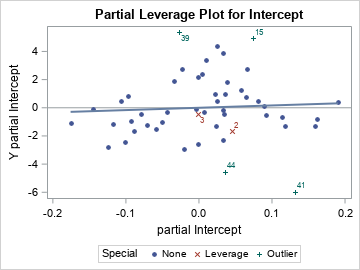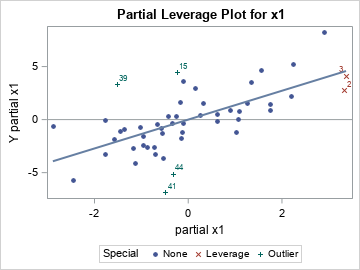The first two plots are shown. The outliers (ID in (15,39,41,44)) will have large residuals in EVERY partial regression plot. In each scatter plot, you can see that the green markers with the "plus" (+) symbol are far from the regression line. Therefore, they are outliers in every scatter plot.

### Identify high-leverage points by using partial regression leverage plots

In a similar way, the partial regression plots enable you to see whether a high-leverage point has extreme values in any partial coordinate. For this example, two high-leverage points are ID=2 and ID=3, and they are displayed as red X-shaped markers.

The previous section showed two partial regression plots. In the partial plot for the Intercept, the two influential observations do not look unusual. However, in the x1 partial plot, you can see that both observations have extreme values (both positive) for the "partial x1" variable.

Let's look at the other two partial regression plots: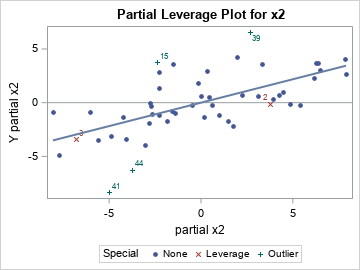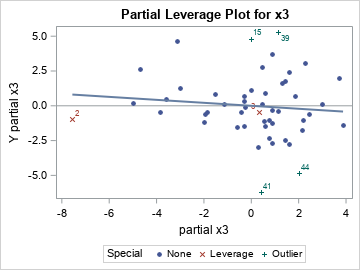The partial regression plot for x2 shows that the "partial x2" coordinate is extreme (very negative) for ID=3. The partial regression plot for x3 shows that the "partial x3" coordinate is extreme (very negative) for ID=2. Remember that these extreme values are not the coordinates of the variables themselves, but of the residuals when you regress each variable onto the other regressors.

It is not easy to explain in a few sentences how the high-leverage points appear in the partial regression plots. I think the details are described in Belsley, Kuh, and Welsch (Regression Diagnostics, 1980), but I cannot check that book right now because I am working from home. But these extreme values are why the Wikipedia article about partial regression plots states, "the influences of individual data values on the estimation of a coefficient are easy to see in this plot."

### Summary

In summary, the partial regression leverage plots provide a way to visualize several important features of a high-dimensional regression problem. The PROC REG documentation includes a brief description of how to create partial regression leverage plots in SAS. As shown in this article, the slope of a partial regression line equals the parameter estimate, and the relative flatness of the line enables you to visualize the null hypothesis βi=0.

This article describes how to interpret the plots to learn more about the regression model. For example, outliers in the full model are also outliers in every partial regression plot. Observations that have small residuals in the full model also have small residuals in every partial regression plot. The high-leverage points will often show up as extreme values in one or more partial regression plots. To examine outliers and high-leverage plots in SAS, you can use the PARTIALDATA option to write the partial regression coordinates to a data set, then add labels for the observations of interest.

Partial regression leverage plots are a useful tool for analyzing the fit of a regression model. They are most useful when the number of observations is not too big. I recommend them when the sample size is 500 or less.

The post Partial leverage plots appeared first on The DO Loop.

An early method for robust regression was iteratively reweighted least-squares regression (Huber, 1964). This is an iterative procedure in which each observation is assigned a weight. Initially, all weights are 1. The method fits a least-squares model to the weighted data and uses the size of the residuals to determine a new set of weights for each observation. In most implementations, observations that have large residuals are downweighted (assigned weights less than 1) whereas observations with small residuals are given weights close to 1. By iterating the reweighting and fitting steps, the method converges to a regression that de-emphasizes observations whose residuals are much bigger than the others.

This form of robust regression is also called M estimation. The "M" signifies that the method is similar to Maximum likelihood estimation. The method tries to minimize a function of the weighted residuals. This method is not robust to high-leverage points because they have a disproportionate influence on the initial fit, which often means they have small residuals and are never downweighted.

The results of the regression depend on the function that you use to weight the residuals. The ROBUSTREG procedure in SAS supports 10 different "weighting functions." Some of these assign a zero weight to observations that have large residuals, which essentially excludes that observation from the fit. Others use a geometric or exponentially decaying function to assign small (but nonzero) weights to large residual values. This article visualizes the 10 weighting functions that are available in SAS software for performing M estimation in the ROBUSTREG procedure. You can use the WEIGHTFUNCTION= suboption to the METHOD=M option on the PROC ROBUSTREG statement to choose a weighting function.

See the documentation of the ROBUSTREG procedure for the formulas and default parameter values for the 10 weighting functions. The residuals are standardized by using a robust estimate of scale. In the rest of this article, "the residual" actually refers to a standardized residual, not the raw residual. The plots of the weighting functions are shown on the interval[-6, 6] and show how functions assign weights based on the magnitude of the standardized residuals.

### Differentiable weighting functions

If you are using iteratively reweighted least squares to compute the estimates, it doesn't matter whether the weighting functions are differentiable. However, if you want to use optimization methods to solve for the parameter estimates, many popular optimization algorithms work best when the objective function is differentiable. The objective function is often related to a sum that involves the weighted residuals, so let's first look at weighting functions that are differentiable. The following four functions are differentiable transformations that assign weights to observations based on the size of the standardized residual.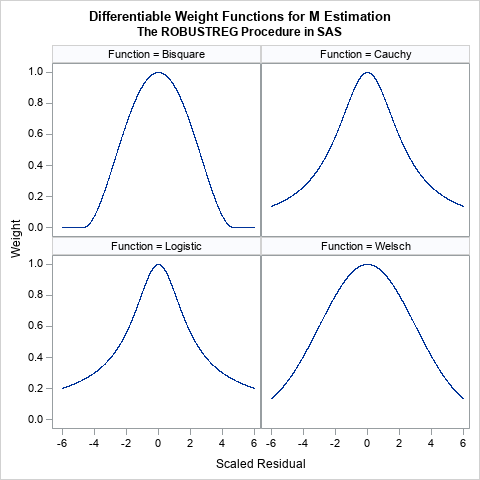These functions all look similar. They are all symmetric functions and look like the quadratic function 1 – a r2 close to r = 0. However, the functions differ in the rate at which they decrease away from r = 0:

• The bisquare function (also called the Tukey function) is a quartic function on the range [-c, c] and equals 0 outside that interval. This is the default weight function for M estimation in PROC ROBUSTREG. The function is chosen so that the derivative at ±c is 0, and thus the function is differentiable. An observation is excluded from the computation if the magnitude of the standardized residual exceeds c. By default, c=4.685.
• The Cauchy and Logistic functions are smooth functions that decrease exponentially. If you use these functions, the weight for an observation is never 0, but the weight falls off rapidly as the magnitude of r increases.
• The Welsch function is a rescaled Gaussian function. The weight for an observation is never 0, but the weight falls off rapidly (like exp(-a r2)) as the magnitude of r increases.

### Nondifferentiable weighting functions

The following six functions are not differentiable at one or more points.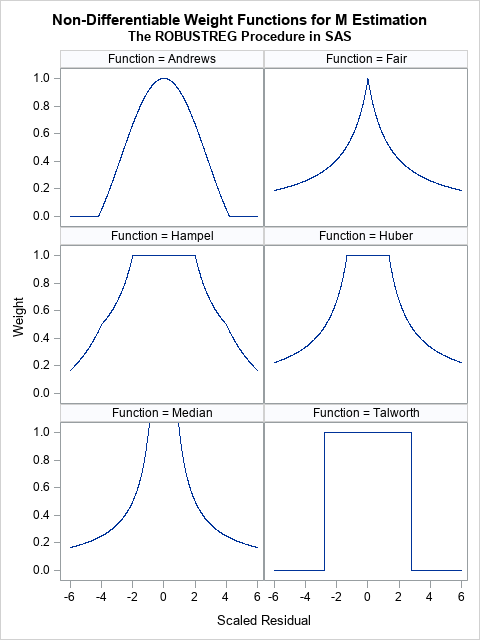• The Andrews function is the function c*sin(r/c)/r within the interval [-c π, c π] and 0 outside. a quartic function on the range [-c, c] and equals 0 outside that interval. It is similar to the bisquare function (see the previous section) but is not differentiable at ±c π.
• The Fair and Median functions are similar. The assign a weight that is asymptotically proportional to 1/|r|. Use the Fair function if you want to downweight observations according to the inverse magnitude of their residual value. Do not use the Median function, which assigns huge weights to observations with small residuals.
• The Hampel, Huber, and Talworth functions are similar because they all assign unit weight to residuals that are small in magnitude. The Talworth function is the most Draconian weight function: it assigns each observation a weight of 1 or 0, depending on whether the magnitude of the residual exceeds some threshold. The Hampel and Huber functions assign a weight of 1 for small residuals, then assign weights in a decreasing manner. If you use the default parameter values for the Hampel function, the weights are exactly 0 outside of the interval [-8, 8].

When I use M estimation, I typically use one of the following three functions:

• The bisquare function, which excludes observations whose residuals have a magnitude greater than 4.685.
• The Welsch function, which gently downweights large residuals.
• The Huber function, which assigns 1 for the weight of small residuals and assigns weights to larger residuals that are proportional to the inverse magnitude.

### Summary

M estimation is a robust regression technique that assigns weights to each observation. The idea is to assign small (or even zero) weights to observations that have large residuals and weights that are close to unity for residuals that are small. (In practice, the algorithm uses standardize residuals to assign weights.) There are many ways to assign weights, and SAS provides 10 different weighting functions. This article visualizes the weighting functions. The results of the regression depend on the function that you use to weight the residuals.

### Appendix: SAS program to visualize the weighting functions in PROC ROBUSTREG

I used SAS/IML to define the weighting functions because the language supports default arguments for functions, whereas PROC FCMP does not. Each function evaluates a vector of input values (x) and returns a vector of values for the weighting function. The default parameter values are specified by using the ARG=value notation in the function signature. If you do not specify the optional parameter, the default value is used.

The following statements produce a 2 x 2 panel of graphs that shows the shapes of the bisquare, Cauchy, logistic, and Welsch functions:

/***********************************/ /* Differentiable weight functions */ /* Assume x is a column vector */ /***********************************/   proc iml; /* The derivarive of Bisquare is f'(x)=4x(x^2-c^2)/c^4, so f'( +/-c )= 0 However, second derivative is f''(x) = 4(3x^2-c^2)/c^4, so f''(c) ^= 0 */ start Bisquare(x, c=4.685); /* Tukey's function */ w = j(nrow(x), 1, 0); /* initialize output */ idx = loc( abs(x) <= c ); if ncol(idx)>0 then do; y = x[idx]/c; w[idx] = (1 - y##2)##2; end; return ( w ); finish;   start Cauchy(x, c=2.385); w = 1 / (1 + (abs(x)/c)##2); return ( w ); finish;   start Logistic(x, c=1.205); w = j(nrow(x), 1, 1); /* initialize output */ idx = loc( abs(x) > constant('maceps') ); /* lim x->0 f(x) = 1 */ if ncol(idx)>0 then do; y = x[idx]/c; w[idx] = tanh(y) / y; end; return ( w ); finish;   start Welsch(x, c=2.985); w = exp( -(x/c)##2 / 2 ); return ( w ); finish;   x = T(do(-6, 6, 0.005)); Function = j(nrow(x), 1, BlankStr(12));   create WtFuncs var {'Function' 'x' 'y'}; y = Bisquare(x); Function[,] = "Bisquare"; append; y = Cauchy(x); Function[,] = "Cauchy"; append; y = Logistic(x); Function[,] = "Logistic"; append; y = Welsch(x); Function[,] = "Welsch"; append; close;   quit;   ods graphics/ width=480px height=480px; title "Differentiable Weight Functions for M Estimation"; title2 "The ROBUSTREG Procedure in SAS"; proc sgpanel data=WtFuncs; label x = "Scaled Residual" y="Weight"; panelby Function / columns=2; series x=x y=y; run;

The following statements produce a 3 x 2 panel of graphs that shows the shapes of the Andrews, Fair, Hampel, Huber, median, and Talworth functions:

/*******************************/ /* Non-smooth weight functions */ /* Assume x is a column vector */ /*******************************/ proc iml;   start Andrews(x, c=1.339); w = j(nrow(x), 1, 0); /* initialize output */ idx = loc( abs(x) <= constant('pi')*c ); if ncol(idx)>0 then do; y = x[idx]/c; w[idx] = sin(y) / y; end; return ( w ); finish;   start Fair(x, c=1.4); w = 1 / (1 + abs(x)/c); return ( w ); finish;   start Hampel(x, a=2, b=4, c=8); w = j(nrow(x), 1, 0); /* initialize output */ idx = loc( abs(x) <= a ); if ncol(idx)>0 then w[idx] = 1; idx = loc( a < abs(x) & abs(x) <= b ); if ncol(idx)>0 then w[idx] = a / abs(x[idx]); idx = loc( b < abs(x) & abs(x) <= c ); if ncol(idx)>0 then w[idx] = (a / abs(x[idx])) # (c - abs(x[idx])) / (c-b); return ( w ); finish;   start Huber(x, c=1.345); w = j(nrow(x), 1, 1); /* initialize output */ idx = loc( abs(x) >= c ); if ncol(idx)>0 then do; w[idx] = c / abs(x[idx]); end; return ( w ); finish;   start MedianWt(x, c=0.01); w = j(nrow(x), 1, 1/c); /* initialize output */ idx = loc( x ^= c ); if ncol(idx)>0 then do; w[idx] = 1 / abs(x[idx]); end; return ( w ); finish;   start Talworth(x, c=2.795); w = j(nrow(x), 1, 0); /* initialize output */ idx = loc( abs(x) < c ); if ncol(idx)>0 then do; w[idx] = 1; end; return ( w ); finish;   x = T(do(-6, 6, 0.01)); Function = j(nrow(x), 1, BlankStr(12)); create WtFuncs var {'Function' 'x' 'y'};   y = Andrews(x); Function[,] = "Andrews"; append; y = Fair(x); Function[,] = "Fair"; append; y = Hampel(x); Function[,] = "Hampel"; append; y = Huber(x); Function[,] = "Huber"; append; y = MedianWt(x); Function[,] = "Median"; append; y = Talworth(x); Function[,] = "Talworth";append; close; quit;   ods graphics/ width=480px height=640px; title "Non-Differentiable Weight Functions for M Estimation"; title2 "The ROBUSTREG Procedure in SAS"; proc sgpanel data=WtFuncs; label x = "Scaled Residual" y="Weight"; panelby Function / columns=2; series x=x y=y; rowaxis max=1; run;

The post Weights for residuals in robust regression appeared first on The DO Loop.

If you are thinking that nobody in their right mind would implement a Calculator API Service with a machine learning model, then yes, you’re probably right. But considering curiosity is in my DNA, it sometimes works this way and machine learning is fun. I have challenged myself to do it, not for the purpose of promoting such an experiment into production, but simply accomplishing a self-challenge that can be easily achieved with the resources provided by SAS Viya, particularly SAS Model Studio and SAS Intelligent Decisioning.

So, first, let’s define the purpose of my challenge:

### deploy a basic calculator API service capable of executing the following operations for two input decimal numbers: addition, subtraction, multiplication, and division

The challenge must be executed under these restrictions:

• Usage of a machine learning model as the “compute-engine” of the calculator
• Development under the low code / no code paradigm
• The end-to-end setup and execution process should not take more than a couple of hours

Use the following tools in SAS Viya:

• Model Studio
• Intelligent Decisioning
• Simple web app (set up not covered)

# The plan

The steps that I am identifying to complete my challenge are:
Step 1 - Create a machine learning model representing the compute-engine of my calculator (Model Studio)
Step 2 - Determine how to process other mathematical operations
Step 3 - Step 3 - Embed the needed logic into the decision to perform the calculator operations (Intelligent Decisioning)
Step 4 - Publish the artifact created as an API service (web app created outside of this post)

## Step 1. Create a machine learning model as the compute-engine

Our first step is to create a model. We start with the addition operation and build from there. We’ll perform the addition by normal means of adding two numbers. Next, we’ll apply some extra logic which will perform subtraction, multiplication, and division. The diagram below represents the process: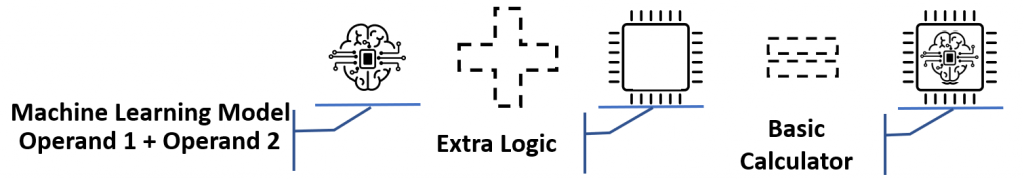A machine learning model is built from a data set where it self-learns what to do. I want my model to learn the addition of two numbers. I created a training data set in Excel with 100 registers, each of them with two random numbers between 0 and 1 and then the sum of them. The image below displays the general setup: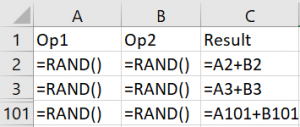The algorithm / model I chose for my compute engine is the linear regression. Linear regression is a simple machine learning algorithm based in the following formula:

y = a + X1 + c·X2 + d·X3 + … + n·Xn

Where:

• y is the result of the model execution – the result of the addition operation
• X1, X2, X3, …, Xn are the input variables – for the calculator, there only will be X1 and X2 as operands
• a, b, c, d, …, n are the parameters the machine learning process determines to create the model

So, with the training data set created, I’ll open a new machine learning project in SAS Viya Model Studio, selecting my data set from where the algorithm will learn, assign the target variable, add linear regression node, a test node, and click “Run pipeline”. Note: if following along in your own environment, make sure to use Selection Method = Adaptive LASSO and toggle Suppress intercept = On in the linear regression node. The resulting model resembles the following: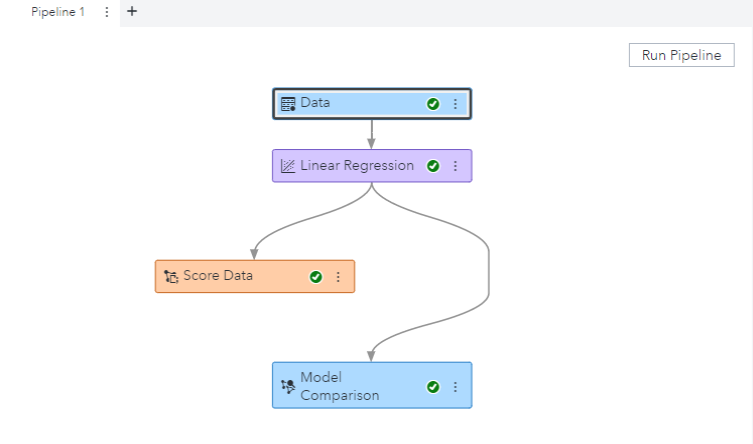Once the pipeline completes, and reviewing the initial results, it seems the model is behaving in a proper way; but when I test specific operands where the result is zero, I realize the model has misgivings: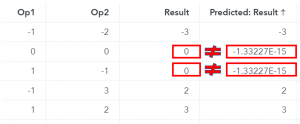Those are the operations with zero as result. I think that maybe, the algorithm hasn’t learned with the proper data, so I change the first seven registers of my initial dataset with the following operations with zeros: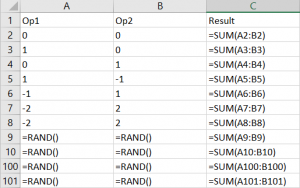Again, running the pipeline and letting the magic work, Voila!!!, the process has learned to handle the zeroes and properly sum two input numbers. When I check the results, I verify the results calculated (predicted) by the model are the same as the original ones that were indicated in the training and test dataset. So now, I am sure my new model is ready for use as my calculator compute engine.

Now that I have my compute engine (model) ready, it’s time to use it. We know it can perform the sum operation perfectly, so how do we perform the rest of the operations? We’ll take the sum model, move it into SAS Intelligent Decisioning, and add rules to handle the rest of the operations.

First, let’s explore the logic that will build the operations from our original model. This is where mathematics start playing in (Step 2). After exploring the operations we'll look at the Decision model where we'll define the logic to run the operations (Step 3).

Execute the model with the two input numbers, with no additional logic.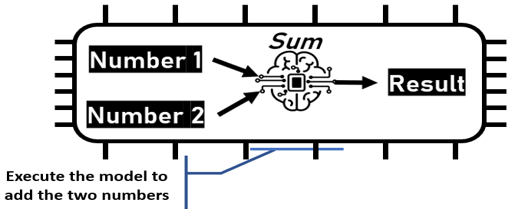### Subtraction

By changing the sign of the second input, the model does the rest.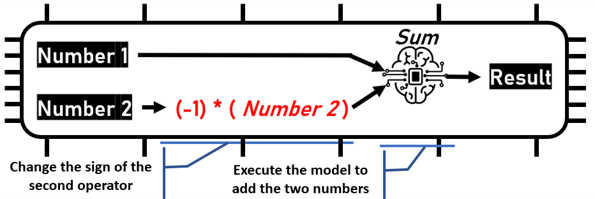That’s a simple enough solution for subtraction, but how do we handle multiplication and division? Let’s take a look.

### Multiplication and division

How can I perform a multiplication or a division operation if my compute engine only executes the addition operation? Here we can apply the magic of logarithmic properties stating:

• log (x*y) = log(x) + log(y)
• log (x/y) = log(x) – log(y)

Following this logic, if I want to multiply two numbers, I calculate the logarithm of each one and perform the addition operation in my compute engine. I follow this up by applying the exponential function to reverse the logarithm. The image below outlines the workflow.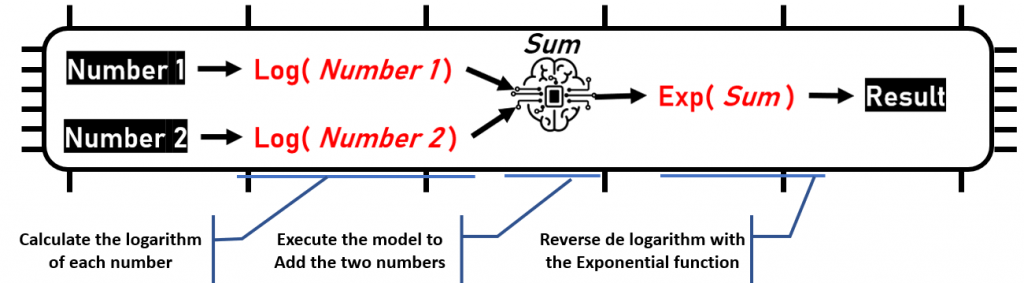For the division, it is the same process, but changing the sign of the second logarithm to the subtraction operation.

There are also certain cases requiring special treatment. For example, a division by zero generates an error, a logarithm of zero or a negative number cannot be calculated, and the multiplication of two numbers is zero if at least one of them is zero.

Let's now build the calculator in SAS Intelligent Decisioning, including the operations, the model, and the extra logic.

## Step 3 - Embed the needed logic into the decision to perform the calculator operations

The diagram below represents the Decision flow for our calculator example. Each node is numbered and followed by a definition.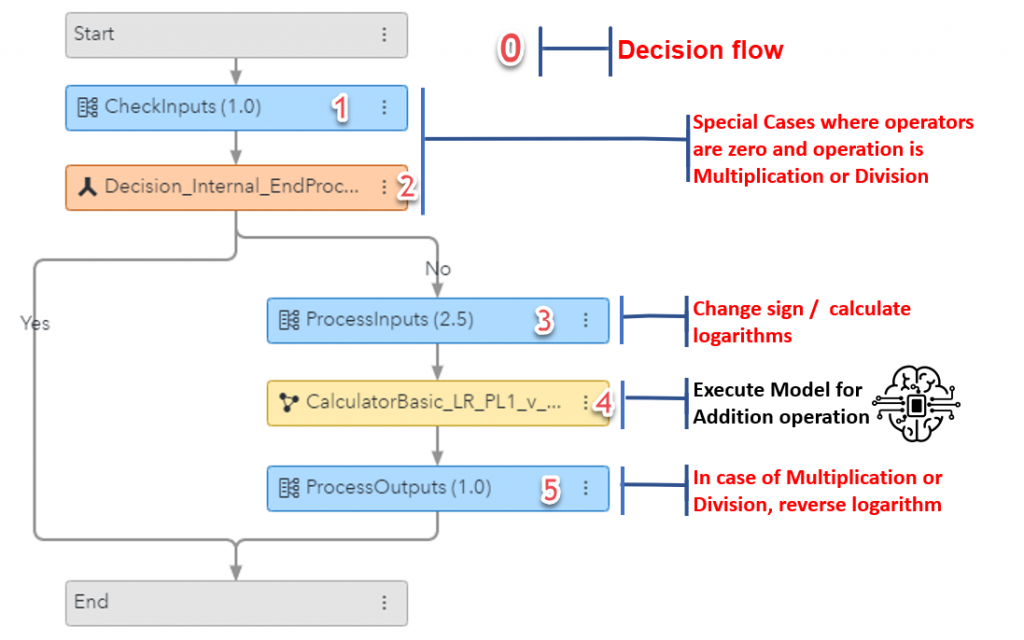0 - Overall decision flow - definition doc found here on GitHub
1 - Determine if zero is a factor for multiplication or division operations - definition doc found here on GitHub
2 - Decision handles value of previous step - set Variable = Decision_Internal_EndProcess = True
3 - Process calculations based on operation value - definition doc found here on GitHub
4 - Calculator linear regression model created earlier in the post - model definition file found here on GitHub
5 - Additional logic to finalize processing on multiplication and division operations - definition doc found here on GitHub

## Step 4 - Publish the final artifact created as an API service

After completing all the work on the decision, click on the Publish button and the Calculator is ready to be consumed via an API.

A colleague of mine created a simple web application which calls models using SAS Viya microservice APIs. I'll use this web app to display the results of my calculator. For brevity, I won't cover the details of the app. If you'd like to see how to create a web app using SAS APIs, I recommend the Build a web application using SAS Compute Server series on the SAS Communities.

The app allows me to choose my decision flow, add my operands and indicate an operation as seen below.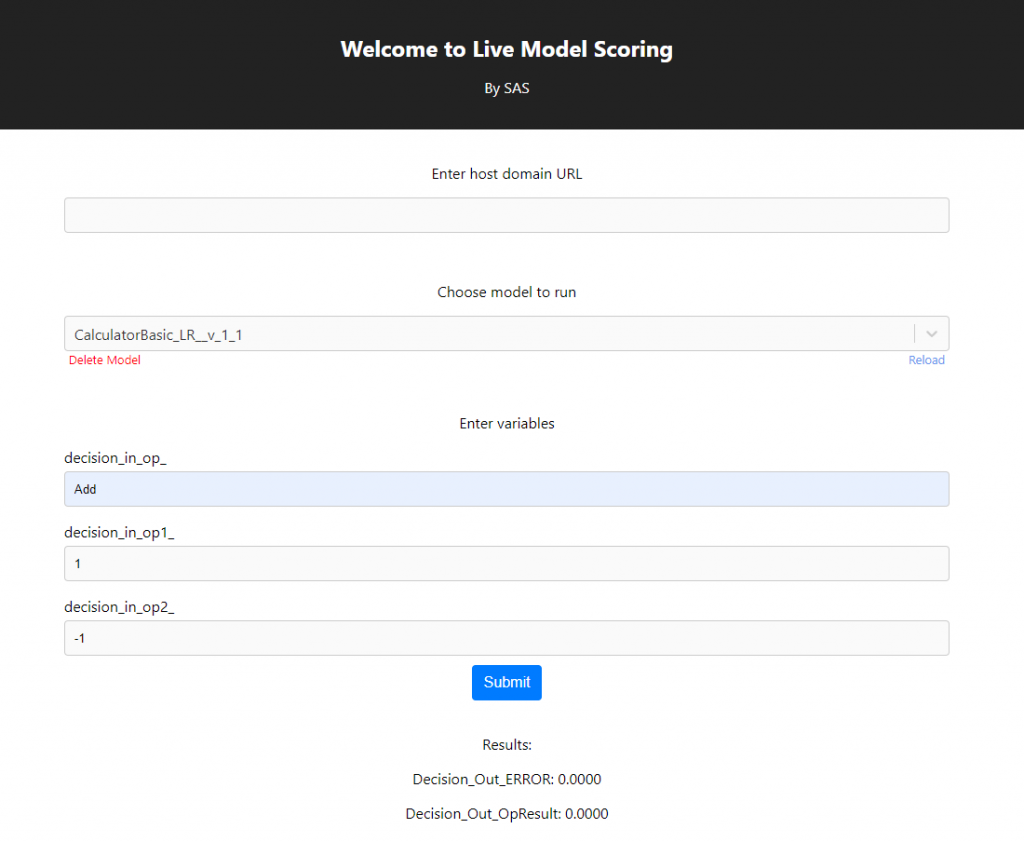I tested with several operand and operation combinations and they all checked out. It worked!

# Final Thoughts

I can consider my self-challenge solved. Through this example we accomplished the following:

• The Calculator API Service can perform the four operations based on a Machine Learning Model.
• I created a simple Machine Learning Model to perform the addition of two decimal numbers from a 100 registers data set.
• The model and the extra logic needed to perform the operations was developed under the low code / no code paradigm.
• I used the visual interface to generate the model and the extra logic, in conjunction with the expression builder, to apply the logarithm and exponential operations.
• The overall process has taken no more than a couple of hours.

Apart from the usefulness of this API, my principal takeaways of this self-challenge are:

• In this case, building my data set to obtain the exact behavior I wanted for my model was quite straight-forward.
• Building the model through the Graphical User Interface was easy and fast.
• Having the capacity to embed the models with extra logic under the low code / no code paradigm provides “supercharged intelligence” to the model
• The publishing feature of the whole artifact as an API service is great, providing instant value to the consumers.
• SAS Viya is a great platform as it provides all the pieces needed to satisfy your analytical business needs as well as your “curiosity needs”.If you are thinking that nobody in their right mind would implement a Calculator API Service with a machine learning model, then yes, you’re probably right. But considering curiosity is in my DNA, it sometimes works this way and machine learning is fun. I have challenged myself to do it, not for the purpose of promoting such an experiment into production, but simply accomplishing a self-challenge that can be easily achieved with the resources provided by SAS Viya, particularly SAS Model Studio and SAS Intelligent Decisioning.

So, first, let’s define the purpose of my challenge:

### deploy a basic calculator API service capable of executing the following operations for two input decimal numbers: addition, subtraction, multiplication, and division

The challenge must be executed under these restrictions:

• Usage of a machine learning model as the “compute-engine” of the calculator
• Development under the low code / no code paradigm
• The end-to-end setup and execution process should not take more than a couple of hours

Use the following tools in SAS Viya:

• Model Studio
• Intelligent Decisioning
• Simple web app (set up not covered)

# The plan

The steps that I am identifying to complete my challenge are:
Step 1 - Create a machine learning model representing the compute-engine of my calculator (Model Studio)
Step 2 - Determine how to process other mathematical operations
Step 3 - Step 3 - Embed the needed logic into the decision to perform the calculator operations (Intelligent Decisioning)
Step 4 - Publish the artifact created as an API service (web app created outside of this post)

## Step 1. Create a machine learning model as the compute-engine

Our first step is to create a model. We start with the addition operation and build from there. We’ll perform the addition by normal means of adding two numbers. Next, we’ll apply some extra logic which will perform subtraction, multiplication, and division. The diagram below represents the process:A machine learning model is built from a data set where it self-learns what to do. I want my model to learn the addition of two numbers. I created a training data set in Excel with 100 registers, each of them with two random numbers between 0 and 1 and then the sum of them. The image below displays the general setup:The algorithm / model I chose for my compute engine is the linear regression. Linear regression is a simple machine learning algorithm based in the following formula:

y = a + X1 + c·X2 + d·X3 + … + n·Xn

Where:

• y is the result of the model execution – the result of the addition operation
• X1, X2, X3, …, Xn are the input variables – for the calculator, there only will be X1 and X2 as operands
• a, b, c, d, …, n are the parameters the machine learning process determines to create the model

So, with the training data set created, I’ll open a new machine learning project in SAS Viya Model Studio, selecting my data set from where the algorithm will learn, assign the target variable, add linear regression node, a test node, and click “Run pipeline”. Note: if following along in your own environment, make sure to use Selection Method = Adaptive LASSO and toggle Suppress intercept = On in the linear regression node. The resulting model resembles the following:Once the pipeline completes, and reviewing the initial results, it seems the model is behaving in a proper way; but when I test specific operands where the result is zero, I realize the model has misgivings:Those are the operations with zero as result. I think that maybe, the algorithm hasn’t learned with the proper data, so I change the first seven registers of my initial dataset with the following operations with zeros:Again, running the pipeline and letting the magic work, Voila!!!, the process has learned to handle the zeroes and properly sum two input numbers. When I check the results, I verify the results calculated (predicted) by the model are the same as the original ones that were indicated in the training and test dataset. So now, I am sure my new model is ready for use as my calculator compute engine.

Now that I have my compute engine (model) ready, it’s time to use it. We know it can perform the sum operation perfectly, so how do we perform the rest of the operations? We’ll take the sum model, move it into SAS Intelligent Decisioning, and add rules to handle the rest of the operations.

First, let’s explore the logic that will build the operations from our original model. This is where mathematics start playing in (Step 2). After exploring the operations we'll look at the Decision model where we'll define the logic to run the operations (Step 3).

Execute the model with the two input numbers, with no additional logic.### Subtraction

By changing the sign of the second input, the model does the rest.That’s a simple enough solution for subtraction, but how do we handle multiplication and division? Let’s take a look.

### Multiplication and division

How can I perform a multiplication or a division operation if my compute engine only executes the addition operation? Here we can apply the magic of logarithmic properties stating:

• log (x*y) = log(x) + log(y)
• log (x/y) = log(x) – log(y)

Following this logic, if I want to multiply two numbers, I calculate the logarithm of each one and perform the addition operation in my compute engine. I follow this up by applying the exponential function to reverse the logarithm. The image below outlines the workflow.For the division, it is the same process, but changing the sign of the second logarithm to the subtraction operation.

There are also certain cases requiring special treatment. For example, a division by zero generates an error, a logarithm of zero or a negative number cannot be calculated, and the multiplication of two numbers is zero if at least one of them is zero.

Let's now build the calculator in SAS Intelligent Decisioning, including the operations, the model, and the extra logic.

## Step 3 - Embed the needed logic into the decision to perform the calculator operations

The diagram below represents the Decision flow for our calculator example. Each node is numbered and followed by a definition.0 - Overall decision flow - definition doc found here on GitHub
1 - Determine if zero is a factor for multiplication or division operations - definition doc found here on GitHub
2 - Decision handles value of previous step - set Variable = Decision_Internal_EndProcess = True
3 - Process calculations based on operation value - definition doc found here on GitHub
4 - Calculator linear regression model created earlier in the post - model definition file found here on GitHub
5 - Additional logic to finalize processing on multiplication and division operations - definition doc found here on GitHub

## Step 4 - Publish the final artifact created as an API service

After completing all the work on the decision, click on the Publish button and the Calculator is ready to be consumed via an API.

A colleague of mine created a simple web application which calls models using SAS Viya microservice APIs. I'll use this web app to display the results of my calculator. For brevity, I won't cover the details of the app. If you'd like to see how to create a web app using SAS APIs, I recommend the Build a web application using SAS Compute Server series on the SAS Communities.

The app allows me to choose my decision flow, add my operands and indicate an operation as seen below.I tested with several operand and operation combinations and they all checked out. It worked!

# Final Thoughts

I can consider my self-challenge solved. Through this example we accomplished the following:

• The Calculator API Service can perform the four operations based on a Machine Learning Model.
• I created a simple Machine Learning Model to perform the addition of two decimal numbers from a 100 registers data set.
• The model and the extra logic needed to perform the operations was developed under the low code / no code paradigm.
• I used the visual interface to generate the model and the extra logic, in conjunction with the expression builder, to apply the logarithm and exponential operations.
• The overall process has taken no more than a couple of hours.

Apart from the usefulness of this API, my principal takeaways of this self-challenge are:

• In this case, building my data set to obtain the exact behavior I wanted for my model was quite straight-forward.
• Building the model through the Graphical User Interface was easy and fast.
• Having the capacity to embed the models with extra logic under the low code / no code paradigm provides “supercharged intelligence” to the model
• The publishing feature of the whole artifact as an API service is great, providing instant value to the consumers.
• SAS Viya is a great platform as it provides all the pieces needed to satisfy your analytical business needs as well as your “curiosity needs”.One of the benefits of using the SWEEP operator is that it enables you to "sweep in" columns (add effects to a model) in any order. This article shows that if you use the SWEEP operator, you can compute a SSCP matrix and use it repeatedly to estimate any linear regression model that uses those effects.

This post relates to a previous post, in which I showed that you never need to use a permutation matrix to rearrange the order of rows and columns of a matrix. As an application, I used the sum of squares and crossproducts (SSCP) matrix, which is used to estimate the regression coefficients in a least-squares regression model. Being able to permute the rows and columns of the SSCP matrix efficiently means that you can solve the linear regression problem very quickly for any ordering of the columns of the design matrix. But if you use the SWEEP operator, you do not need to permute the SSCP matrix at all!

### The SSCP matrix in PROC REG

Before discussing the SWEEP operator, let's review a little-used feature of PROC REG in SAS. The REG procedure is interactive, which means that you can interactively add or delete effects from a model as part of the model-building process. However, if you are going to explore several different models, you must use the VAR statement to specify all variables that you might conceivably want to use BEFORE the first RUN statement. Why? Because when PROC REG encounters the RUN statement it builds the SSCP matrix for the variables that you have specified. As stated in the documentation, for each subsequent model, "the procedure selects the appropriate crossproducts from the [SSCP] matrix." In other words, PROC REG re-uses that SSCP matrix for every MODEL statement.

Let's look at an example. The following call to PROC REG computes the SSCP matrix for five variables and the intercept effect. It uses that SSCP matrix to compute the parameter estimates:

proc reg data=sashelp.cars plots=NONE; ods select ParameterEstimates; VAR MSRP EngineSize Horsepower MPG_Highway Weight; /* vars in the SSCP */ FULL: model MSRP = EngineSize Horsepower MPG_Highway Weight; /* uses the SSCP */ run;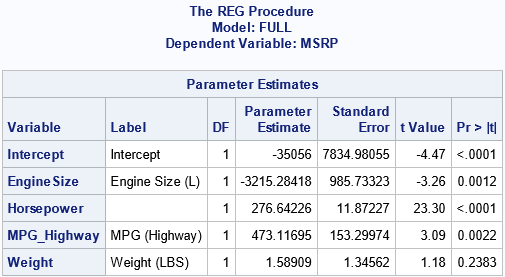Because PROC REG is an interactive procedure, the procedure does not exit when it encounters the RUN statement. It remembers the SSCP matrix until the procedure exits. If you submit additional MODEL statements, PROC REG will use the SSCP matrix to estimate the new parameters, as long as the variables were previously specified. For example, you can specify the same model, but list the variables in a different order. Or you can estimate a reduced model that contains only a subset of the variables, as follows:

 PERMUTE: model MSRP = Horsepower Weight EngineSize MPG_Highway; run; PARTIAL: model MSRP = Weight Horsepower; run;quit;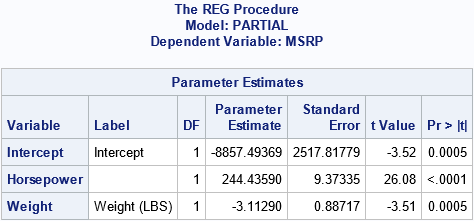The output for the "PERMUTE" model is not shown, because the estimates are the same as for the "FULL" model, but in a different order. It is important to note that PROC REG estimates the second and third models without re-reading the data. It simply re-uses the original SSCP matrix. As I have previously shown, computing the SSCP matrix is often 90% of the work of computing regression estimates, which means that the second and third models are computed very quickly.

The next section shows that how the SWEEP operator can quickly compute the parameter estimates for any model and for any order of the variables. There is no need to physically permute the rows and columns of the SSCP matrix.

### The SWEEP operator

You can use the SAS/IML language to illuminate how PROC REG can compute one SSCP matrix and re-use it for all subsequent models. The following SAS/IML statements read the data and compute the SSCP matrix. Following Goodnight (1979), the last column contains the response variable, but this is merely a convenience.

proc iml; varNames = {'EngineSize' 'Horsepower' 'MPG_Highway' 'Weight'}; use sashelp.cars; read all var varNames into X; read all var 'MSRP' into Y; close; /* add intercept column and response variable */ X = j(nrow(X), 1, 1) || X; /* the design matrix */ k = ncol(X); /* number of effects */   varNames = 'Intercept' || varNames || 'MSRP'; XY = X || Y; SSCP = XY * XY; /* the only SSCP that we'll need */   /* by using the sweep operator (1st row=mean, last col=param est*/ S = sweep(SSCP, 1:k); rCol = nrow(SSCP); /* the response column */ b = S[1:k, rCol]; /* parameter estimates for regression coefficients */ print b[r=varNames];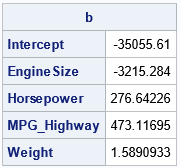The parameter estimates are the same as computed by PROC REG. They were obtained by sweeping the columns of the SSCP matrix in the order 1, 2, ..., 5. You can think of the vector v=1:k as the "identity permutation" of the rows. Then the SWEEP operator for the variables in their original order is the operation S = sweep(SSCP, v).

The SWEEP function in SAS/IML enables you to specify any vector of indices as the second argument. In this way, you can sweep the columns of the SSCP matrix in any order. For example, the "PERMUTE" model from the PROC REG example corresponds to sweeping the SSCP matrix in the order v = {1 3 5 2 4}. As the following example shows, you get the same parameter estimates because the models are the same:

/* sweep original SSCP in the order of v */ v = {1 3 5 2 4}; pVarNames = varNames[,v]; /* effect names in permuted order */ S = sweep(SSCP, v); /* sweep the specified rows in the specified order */ b = S[v, rCol]; /* get the parameter estimates in new order */ print b[r=pVarNames];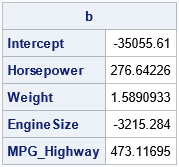As expected, the parameter estimates are the same, but the order of the parameters is different.

You can also estimate parameters for any model that uses a subset of the columns. For example, the model that has the explanatory variables Weight and Horsepower (the fifth and third effects) is computed from the SSCP matrix by sweeping the columns in the order {1 5 3}, as follows:

/* Perform a partial sweep for the model {1 5 3}. No need to form a new design matrix or to extract a portion of the SCCP. */ v = {1 5 3}; pVarNames = varNames[,v]; /* effect names for this model */ S = sweep(SSCP, v); /* sweeps in the variables in the order of v */ b = S[v, rCol]; /* get the parameter estimates */ print b[r=pVarNames];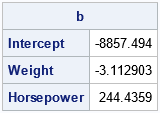The output matches the "PARTIAL" model from PROC REG.

### Summary

In a previous post, I showed an efficient way to permute the order of rows and columns of a matrix. But the current article shows that you do not need to permute the elements of an SSCP matrix if you use the SWEEP operator. The SWEEP operator enables you to specify the order in which you want to add effects to the model. From a design matrix, you can compute the SSCP matrix. (This is most of the work!) You can then quickly compute any model that uses the columns of the design matrix in any order.

This useful property of the SWEEP operator is among the many features discussed in Goodnight, J. (1979), "A Tutorial on the SWEEP Operator," The American Statistician. For other references, see the end of my previous article about the SWEEP operator.

The post More on the SWEEP operator for least-square regression models appeared first on The DO Loop.In a previous article, I discussed various ways to solve a least-square linear regression model. I discussed the SWEEP operator (used by many SAS regression routines), the LU-based methods (SOLVE and INV in SAS/IML), and the QR decomposition (CALL QR in SAS/IML). Each method computes the estimates for the regression coefficients, b, by using the normal equations (XX) b = Xy, where X is a design matrix for the data.

This article describes a QR-based method that does not use the normal equations but works directly with the overdetermined system X b = y. It then compares the performance of the direct QR method to the computational methods that use the normal equations.

### The QR solution of an overdetermined system

As shown in the previous article, you can use the QR algorithm to solve the normal equations. However, if you search the internet for "QR algorithm and least squares," you find many articles that show how you can use the QR decomposition to directly solve the overdetermined system X b = y. How does the direct QR method compare to the methods that use the normal equations?

Recall that X is an n x m design matrix, where n > m and X is assumed to be full rank of m. For simplicity, I will ignore column pivoting. If you decompose X = QRL, the orthogonal matrix Q is n x n, but the matrix RL is not square. ("L" stands for "long.") However, RL is the vertical concatenation of a square triangular matrix and a rectangular matrix of zeros:
${\bf R_L} = \begin{bmatrix} {\bf R} \\ {\bf 0} \end{bmatrix}$
If you let Q1 be the first m columns of Q and let Q2 be the remaining (n-m) columns, you get a partitioned matrix equation:
$\begin{bmatrix} {\bf Q_1} & {\bf Q_2} \end{bmatrix} \begin{bmatrix} {\bf R} \\ {\bf 0} \end{bmatrix} {\bf b} = {\bf y}$
If you multiply both sides by Q (the inverse of the orthogonal matrix, Q), you find out that the important matrix equation to solve is ${\bf R b} = {\bf Q_1^{\prime} y}$. The vector ${\bf Q_1^{\prime} y}$ is the first m rows of the vector ${\bf Q^{\prime} y}$. The QR call in SAS/IML enables you to obtain the triangular R matrix and the vector Q y directly from the data matrix and the observed vector. The following program uses the same design matrix as for my previous article. Assuming X has rank m, the call to the QR subroutine returns the m x m triangular matrix, R, and the vector Q y. You can then extract the first m rows of that vector and solve the triangular system, as follows:

/* Use PROC GLMSELECT to write a design matrix */ proc glmselect data=Sashelp.Class outdesign=DesignMat; class Sex; model Weight = Height Sex Height*Sex/ selection=none; run;   proc iml; use DesignMat; read all var {'Intercept' 'Height' 'Sex_F' 'Height_Sex_F'} into X; read all var {'Weight'} into Y; close;   /* The QR algorithm can work directly with the design matrix and the observed responses. */ call QR(Qty, R, piv, lindep, X, , y); /* return Q*y and R (and piv) */ m = ncol(X); c = QTy[1:m]; /* we only need the first m rows of Q*y */ b = trisolv(1, R, c, piv); /* solve triangular system */ print b[L="Direct QR" F=D10.4];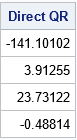This is the same least-squares solution that was found by using the normal equations in my previous article.

### Compare the performance of least-squares solutions

How does this direct method compare with the methods that use the normal equations? You can download a program that creates simulated data and runs each algorithm to estimate the least-squares regression coefficients. The simulated data has 100,000 observations; the number of variables is chosen to be m={10, 25, 50, 75, 100, 250, 500}. The program uses SAS/IML 15.1 on a desktop PC to time the algorithms. The results are shown below: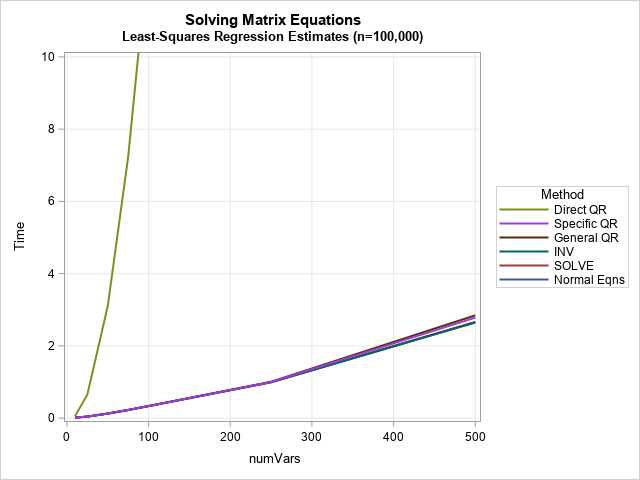The most obvious feature of the graph is that the "Direct QR" method that is described in this article is not as fast as the methods that use the normal equations. For 100 variables and 100,000 observations, the "Direct QR" call takes more than 12 seconds on my PC. (It's faster on a Linux server). The graph shows that the direct method shown in this article is not competitive with the normal-equation-based algorithms when using the linear algebra routines in SAS/IML 15.1.

The graph shows that the algorithms that use the normal equations are relatively faster. For the SAS/IML calls on my PC, you can compute the regression estimates for 500 variables in about 2.6 seconds. The graph has a separate line for the time required to form the normal equations (which you can think of as forming the XX matrix). Most of the time is spent computing the normal equations; only a fraction of the time is spent actually solving the normal equations. The following table shows computations on my PC for the case of 500 variables: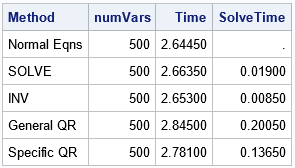The table shows that it takes about 2.6 seconds to compute the XX matrix and the vector Xy. After you form the normal equations, solving them is very fast. For this example, the SOLVE and INV methods take only a few milliseconds to solve a 500 x 500. The QR algorithms take 0.1–0.2 seconds longer. So, for this example, forming the normal equations accounts for more than 90% of the total time.

### SAS regression procedures

These results are not the best that SAS can do. SAS/IML is a general-purpose tool. SAS regression procedures like PROC REG are optimized to compute regression estimates even faster. They also use the SWEEP operator, which is faster than the SOLVE function. For more than 20 years, SAS regression procedures have used multithreaded computations to optimize the performance of regression computations (Cohen, 2002). More recently, SAS Viya added the capability for parallel processing, which can speed up the computations even more. And, of course, they compute much more than only the coefficient estimates! They also compute standard errors, p-values, related statistics (MSE, R square,....), diagnostic plots, and more.

### Summary

This article compares several methods for obtaining least-squares regression estimates. It uses simulated data where the number of observations is much greater than the number of variables. It shows that methods that use the normal equations are faster than a "Direct QR" method, which does not use the normal equations. When you use the normal equations, most of the time is spent actually forming the normal equations. After you have done that, the time required to solve the system is relatively fast.

The post Compare computational methods for least squares regression appeared first on The DO Loop.In computational statistics, there are often several ways to solve the same problem. For example, there are many ways to solve for the least-squares solution of a linear regression model. A SAS programmer recently mentioned that some open-source software uses the QR algorithm to solve least-squares regression problems and asked how that compares with SAS. This article shows how to estimate a least-squares regression model by using the QR method in SAS. It shows how to use the QR method in an efficient way.

### Solving the least-squares problem

Before discussing the QR method, let's briefly review other ways to construct a least-squares solution to a regression problem. In a regression problem, you have an n x m data matrix, X, and an n x 1 observed vector of responses, y. When n > m, this is an overdetermined system and typically there is no exact solution. Consequently, the goal is to find the m x 1 vector of regression coefficients, b, such that the predicted values (X b) are as close as possible to the observed values. If b is a least-squares solution, then b minimizes the vector norm || X b - y ||2, where ||v||2 is the sum of the squares of the components of a vector.

Using calculus, you can show that the solution vector, b, satisfies the normal equations (XX) b = Xy. The normal equations have a unique solution when the crossproduct matrix XX is nonsingular. Most numerical algorithms for least-squares regression start with the normal equations, which have nice numerical properties that can be exploited.

### Creating a design matrix

The first step of solving a regression problem is to create the design matrix. For continuous explanatory variables, this is easy: You merely append a column of ones (the intercept column) to the matrix of the explanatory variables. For more complicated regression models that contain manufactured effects such as classification effects, interaction effects, or spline effects, you need to create a design matrix. The GLMSELECT procedure is the best way to create a design matrix for fixed effects in SAS. The following call to PROC GLMSELECT writes the design matrix to the DesignMat data set. The call to PROC REG estimates the regression coefficients:

proc glmselect data=Sashelp.Class outdesign=DesignMat; /* write design matrix */ class Sex; model Weight = Height Sex Height*Sex/ selection=none; run; /* test: use the design matrix to estimate the regression coefficients */ proc reg data=DesignMat plots=none; model Weight = Height Sex_F Height_Sex_F; run;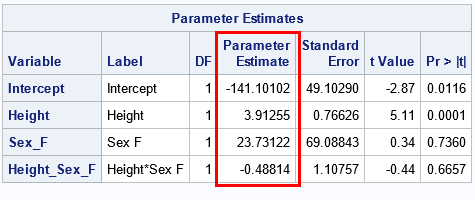The goal of the rest of this article is to reproduce the regression estimates by using various other linear algebra operations. For each method, we want to produce the same estimates: {-141.10, 3.91, 23.73, -0.49}.

### Linear regression: A linear algebraic approach

I've previously discussed the fact that most SAS regression procedures use the sweep operator to construct least-squares solutions. Another alternative is to use the SOLVE function in SAS/IML:

proc iml; use DesignMat; read all var {'Intercept' 'Height' 'Sex_F' 'Height_Sex_F'} into X; read all var {'Weight'} into Y; close;   /* form normal equations */ XpX = X*X; Xpy = X*y;   /* an efficient numerical solution: solve a particular RHS */ b = solve(XpX, Xpy); print b[L="SOLVE" F=D10.4];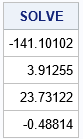The SOLVE function is very efficient and gives the same parameter estimates as the SWEEP operator (which was used by PROC REG). Using the SOLVE function on the system A*b=z is mathematically equivalent to using the matrix inverse to find b=A-1z. However, the INV function explicitly forms the inverse matrix, whereas the SOLVE function does not. Consequently, the SOLVE function is faster and more efficient than using the following SAS/IML statement:

/* a less efficient numerical solution */ Ainv = inv(XpX); /* explicitly form the (m x m) inverse matrix */ b = Ainv*Xpy; /* apply the inverse matrix to RHS */ print b[L="INV" F=D10.4];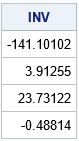For a performance comparison of the SOLVE and INV functions, see the article, "Solving linear systems: Which technique is fastest?"

### An introduction to the QR method for solving linear equations

There are many ways to solve the normal equations. The SOLVE (and INV) functions use the LU decomposition. An alternative is the QR algorithm, which is slower but can be more accurate for ill-conditioned systems. You can apply the QR decomposition to the normal equations or to the original design matrix. This section discusses the QR decomposition of the design matrix. A subsequent article discusses decomposing the data matrix directly.

Let's see how the QR algorithm solves the normal equations. You can decompose the crossproduct matrix as the product of an orthogonal matrix, Q, and an upper-triangular matrix, R. If A = XX, then A = QR. (I am ignoring column pivoting, which is briefly discussed below.) The beauty of an orthogonal matrix is that the transpose equals the inverse. This means that you can reduce the system A b = (X y) to a triangular system R b = Q (X y), which is easily solved by using the TRISOLV function in SAS/IML.

The SAS/IML version of the QR algorithm is a version known as "QR with column pivoting." Using column pivoting improves solving rank-deficient systems and provides better numerical accuracy. However, when the algorithm uses column pivoting, you are actually solving the system AP = QR, where P is a permutation matrix. This potentially adds some complexity to dealing with the QR algorithm. Fortunately, the TRISOLVE function supports column pivoting. If you use the TRISOLV function, you do not need to worry about whether pivoting occurred or not.

### Using the QR method in SAS/IML

Recall from the earlier example that it is more efficient to use the SOLVE function than the INV function. The reason is that the INV function explicitly constructs an m x m matrix, which then is multiplied with the right-hand side (RHS) vector to obtain the answer. In contrast, the SOLVE function never forms the inverse matrix. Instead, it directly applies transformations to the RHS vector.

The QR call in SAS/IML works similarly. If you do not supply a RHS vector, v, then the QR call returns the full m x m matrix, Q. You then must multiply Q v yourself. If you do supply the RHS vector, then the QR call returns Q v without ever forming Q. This second method is more efficient,

See the documentation of the QR call for the complete syntax. The following call uses the inefficient method in which the Q matrix is explicitly constructed:

/* It is inefficient to factor A=QR and explicitly solve the equation R*b = Q*c */ call QR(Q, R, piv, lindep, XpX); /* explicitly form the (m x m) matrix Q */ c = Q*Xpy; /* apply the inverse Q matrix to RHS */ b = trisolv(1, R, c, piv); /* equivalent to b = inv(R)*Q*c */ print b[L="General QR" F=D10.4];

In contrast, the next call is more efficient because it never explicitly forms the Q matrix:

/* It is more efficient to solve for a specific RHS */ call QR(c, R, piv, lindep, XpX, ,Xpy); /* returns c = Q*Xpy */ b = trisolv(1, R, c, piv); /* equivalent to b = inv(R)*Q*c */ print b[L="Specific QR" F=D10.4];

The output is not shown, but both calls produce estimates for the regression coefficients that are exactly the same as for the earlier examples. Notice that the TRISOLV function takes the pivot vector (which represents a permutation matrix) as the fourth argument. That means you do not need to worry about whether column pivoting occurred during the QR algorithm.

### QR applied to the design matrix

As mentioned earlier, you can also apply the QR algorithm to the design matrix, X, and the QR algorithm will return the least-square solution without ever forming the normal equations. This is shown in a subsequent article, which also compares the speed of the various methods for solving the least-squares problem.

### Summary

This article discusses three ways to solve a least-squares regression problem. All start by constructing the normal equations: (XX) b = X y. The solution of the normal equations (b) is the vector that minimizes the squared differences between the predicted values, X b, and the observed responses, y. This article discusses

• the SWEEP operator, which is used by many SAS regression procedures
• the SOLVE and INV function, which use the LU factorization
• the QR call, which implements the QR algorithm with column pivoting

A follow-up article compares the performance of these methods and of another QR algorithm, which does not use the normal equations.

The post The QR algorithm for least-squares regression appeared first on The DO Loop.A previous article discusses how to interpret regression diagnostic plots that are produced by SAS regression procedures such as PROC REG. In that article, two of the plots indicate influential observations and outliers. Intuitively, an observation is influential if its presence changes the parameter estimates for the regression by "more than it should." Various researchers have developed statistics that give a precise meaning to "more than it should," and the formulas for several of the popular influence statistics are included in the PROC REG documentation.

This article discusses the Cook's D and leverage statistics. Specifically, how can you use the information in the diagnostic plots to identify which observations are influential? I show two techniques for identifying the observations. The first uses a DATA step and a formula to identify influential observations. The second technique uses the ODS OUTPUT statement to extract the same information directly from a regression diagnostic plot.

These are not the only regression diagnostic plots that can identify influential observations:

• The DFBETAS statistic estimates the effect that deleting each observation has on the estimates for the regression coefficients.
• The DFFITS statistic is a measure of how the predicted value changes when an observation is deleted. It is closely related to the Cook's D statistic.

### The data and model

As in the previous article, let's use a model that does NOT fit the data very well, which makes the diagnostic plots more interesting. The following DATA step adds a quadratic effect to the Sashelp.Thick data and also adds a variable that is used in a subsequent section to merge the data with the Cook's D and leverage statistics.

data Thick2; set Sashelp.Thick; North2 = North**2; /* add quadratic effect */ Observation = _N_; /* for merging with other data sets */ run;

### Graphs and labels

Rather than create the entire panel of diagnostic plots, you can use the PLOTS(ONLY)= option to create only the graphs for Cook's D statistic and for the studentized residuals versus the leverage. In the following call to PROC REG, the LABEL suboption requests that the influential observations be labeled. By default, the labels are the observation numbers. You can use the ID statement in PROC REG to specify a variable to use for the labels.

proc reg data=Thick2 plots(only label) =(CooksD RStudentByLeverage); model Thick = North North2 East; /* can also use INFLUENCE option */ run;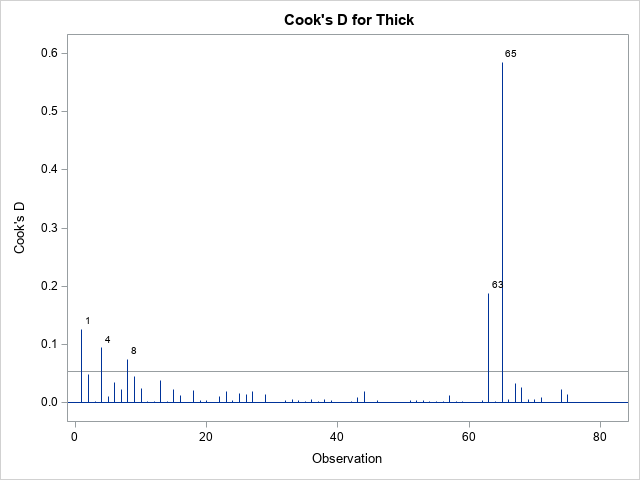The graph of the Cook'd D statistic is shown above. The PROC REG documentation states that the horizontal line is the value 4/n, where n is the number of observations in the analysis. For these data, n = 75. According to this statistic, observations 1, 4, 8, 63, and 65 are influential.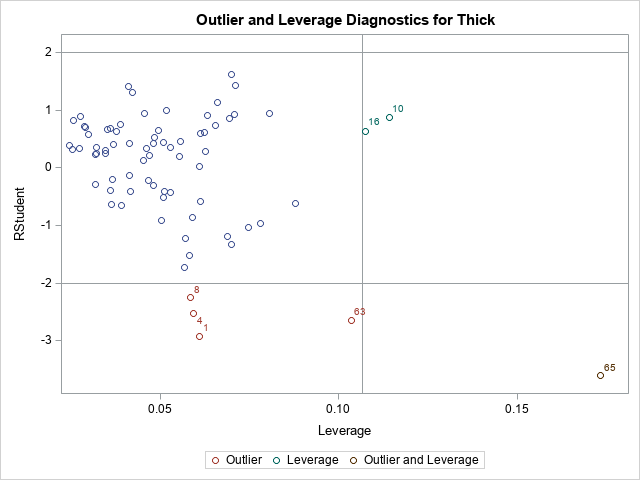The second graph is a plot of the studentized residual versus the leverage statistic. The PROC REG documentation states that the horizontal lines are the values ±2 and the vertical line is at the value 2p/n, where p is the number of parameters, including the intercept. For this model, p = 4. The observations 1, 4, 8, 10, 16, 63, and 65 are shown in this graph as potential outliers or potential high-leverage points.

From the graphs, we know that certain observations are influential. But how can highlight those influential observations in plots, print them, or otherwise analyze them? And how can we automate the process so that it works for any model on any data set?

### Manual computation of influential observations

There are two ways to determine which observations have large residuals or are high-leverage or have a large value for the Cook's D statistic. The traditional way is to use the OUTPUT statement in PROC REG to output the statistics, then identify the observations by using the same cutoff values that are shown in the diagnostic plots. For example, the following DATA step lists the observations whose Cook's D statistic exceeds the cutoff value 4/n ≈ 0.053.

/* manual identification of influential observations */ proc reg data=Thick2 plots=none; model Thick = North North2 East; /* can also use INFLUENCE option */ output out=RegOut predicted=Pred student=RStudent cookd=CookD H=Leverage; quit;   %let p = 4; /* number of parameter in model, including intercept */ %let n = 75; /* Number of Observations Used */ title "Influential (Cook's D)"; proc print data=RegOut; where CookD > 4/&n; var Observation East North Thick CookD; run;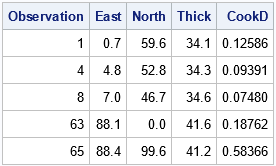This technique works well. However, it assumes that you can easily write a formula to identify the influential observations. This is true for regression diagnostics. However, you can imagine a more complicated graph for which "special" observations are not so easy to identify. As shown in the next section, you can leverage (pun intended) the fact that SAS already identified the special observations for you.

### Leveraging the power of the ODS OUTPUT statement

Did you know that you can create a data set from any SAS graphic? Many SAS programmers use ODS OUTPUT to save a table to a SAS data set, but the same technique enables you to save the data underlying any ODS graph. There is a big advantage of using ODS OUTPUT to get to the data in a graph: SAS has already done the work to identify and label the important points in the graph. You don't need to know any formulas!

Let's see how this works by extracting the observations whose Cook's D statistic exceeds the cutoff value. First, generate the graphs and use ODS OUTPUT to same the underlying data models, as follows:

/* Let PROC REG do the work. Use ODS OUTPUT to capture information */ ods exclude all; proc reg data=Thick2 plots(only label) =(CooksD RStudentByLeverage); model Thick = North North2 East; ods output CooksDPlot=CookOut /* output the data for the Cook's D graph */ RStudentByLeverage=RSOut; /* output the data for the outlier-leverage plot */ quit; ods exclude none;   /* you need to look at the data! */ proc print data=CookOut(obs=12); where Observation > 0; run;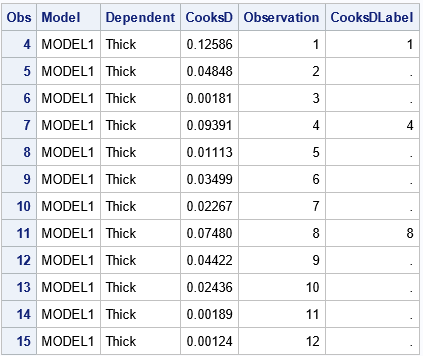When you output the data from a graph, you have to look at how the data are structured. Sometimes the data set has strange variable names or extra observations. In this case, the data begins with three extra observations for which the Cook's D statistic is missing. For the curious, fake observations are often used to set axes ranges or to specify the order of groups in a plot.

Notice that the CookOut data set includes a variable named Observation, which you can use to merge the CookOut data and the original data.

From the structure of the CookOut data set, you can infer that the influential observations are those for which the CooksDLabel variable is nonmissing (excepting the fake observations at the top of the data). Therefore, the following DATA step merges the output data sets and the original data. In the same DATA step, you can create other useful variables, such as a binary variable that indicates which observations have a large Cook's D statistic:

data All; merge Thick2 CookOut RSOut; by Observation; /* create a variable that indicates whether the obs has a large Cook's D stat */ CooksDInf = (^missing(CooksD) & CooksDLabel^=.); label CooksDInf = "Influential (Cook's D)"; run;   proc print data=All noobs; where CooksDInf > 0; var Observation East North Thick; run;   proc sgplot data=All; scatter x=East y=North / group=CooksDInf datalabel=CooksDLabel markerattrs=(symbol=CircleFilled size=10); run;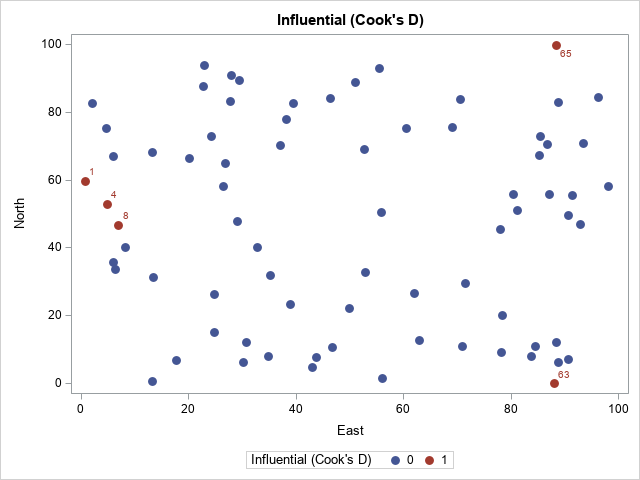The output from PROC PRINT (not shown) confirms that observations 1, 4, 8, 63, and 65 have a large Cook's D statistic. The scatter plot shows that the influential observations are located at extreme values of the explanatory variables.

### Outliers and high-leverage points

The process to extract or visualize the outliers and high-leverage points is similar. The RSOut data set contains the relevant information. You can do the following:

1. Look at the names of the variables and the structure of the data set.
2. Merge with the original data by using the Observation variable.
3. Use one of more variables to identify the special observations.

The following call to PROC PRINT gives you an overview of the data and its structure:

/* you need to look at the data! */ proc print data=RSOut(obs=12) noobs; where Observation > 0; var Observation RStudent HatDiagonal RsByLevIndex outLevLabel RsByLevGroup; run;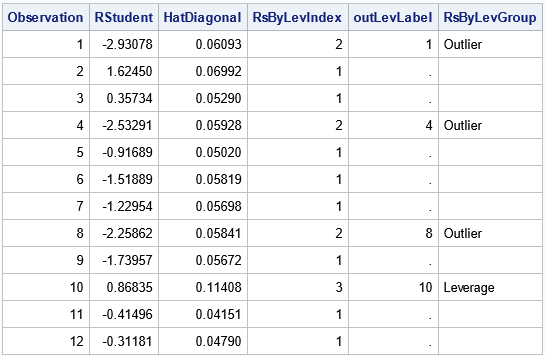For the RSOut data set, the indicator variable is named RsByLevIndex, which has the value 1 for ordinary observations and the value 2, 3, or 4 for influential observations. The meaning of each index value is shown in the RsByLevGroup variable, which has the corresponding values "Outlier," "Leverage," and "Outlier and Leverage" (or a blank string for ordinary observations). You can use these values to identify the outliers and influential observations. For example, you can print all influential observations or you can graph them, as shown in the following statements:

proc print data=All noobs; where Observation > 0 & RsByLevIndex > 1; var Observation East North Thick RsByLevGroup; run;   proc sgplot data=All; scatter x=East y=North / markerattrs=(size=9); /* ordinary points are not filled */ scatter x=East y=North / group=RsByLevGroup nomissinggroup /* special points are filled */ datalabel=OutLevLabel markerattrs=(symbol=CircleFilled size=10); run;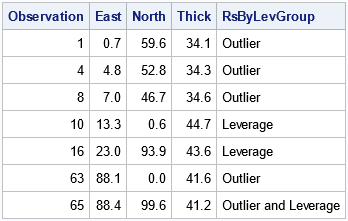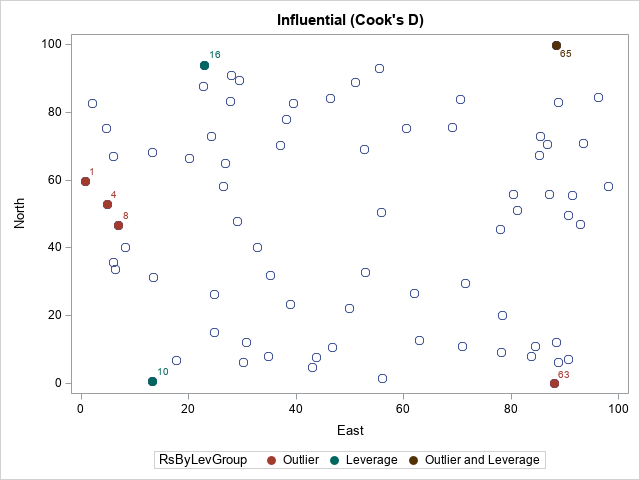The PROC PRINT output confirms that we can select the noteworthy observations. In the scatter plot, the color of each marker indicates whether the observation is an outlier, a high-leverage point, both, or neither.

### Summary

It is useful to identify and visualize outliers and influential observations in a regression model. One way to do this is to manually compute a cutoff value and create an indicator variable that indicates the status of each observation. This article demonstrates that technique for the Cook's D statistic. However, an alternative technique is to take advantage of the fact that SAS can create graphs that label the outliers and influential observations. You can use the ODS OUTPUT statement to capture the data underlying any ODS graph. To visualize the noteworthy observations, you can merge the original data and the statistics, indicator variables, and label variables.

Although it's not always easy to decipher the variable names and the structure of the data that comes from ODS graphics, this technique is very powerful. Its use goes far beyond the regression example in this article. The technique enables you to incorporate any SAS graph into a second analysis or visualization.

The post Identify influential observations in regression models appeared first on The DO Loop.When you fit a regression model, it is useful to check diagnostic plots to assess the quality of the fit. SAS, like most statistical software, makes it easy to generate regression diagnostics plots. Most SAS regression procedures support the PLOTS= option, which you can use to generate a panel of diagnostic plots. Some procedures (most notably PROC REG and PROC LOGISTIC) support dozens of graphs that help you to evaluate the fit of the model, to determine whether the data satisfy various assumptions, and to identify outliers and high-leverage points. Diagnostic plots are most useful when the size of the data is not too large, such as less than 5,000 observations.

This article shows how to interpret diagnostic plots for a model that does not fit the data. For an ill-fitting model, the diagnostic plots should indicate the lack of fit.

This article discusses the eight plots in the DiagnosticsPanel plot, which is produced by several SAS regression procedures. To make the discussion as simple as possible, this article uses PROC REG to fit an ordinary least squares model to the data.

The eight plots can be classified into five groups:

1. A plot of the observed versus predicted responses. (Center of panel.)
2. Two graphs of residual values versus the predicted responses. (Upper left of panel.)
3. Two graphs that indicate outliers, high-leverage observations, and influential observations. (Upper right of panel.)
4. Two graphs that assess the normality of the residuals. (Lower left of panel.)
5. A plot that compares the cumulative distributions of the centered predicted values and the residuals. (Bottom of panel.)

This article also includes graphs of the residuals plotted against the explanatory variables.

### Create a model that does not fit the data

This section creates a regression model that (intentionally) does NOT fit the data. The data are 75 locations and measurements of the thickness of a coal seam. A naive model might attempt to fit the thickness of the coal seam as a linear function of the East-West position (the East variable) and the South-North position (the North variable). However, even a cursory glance at the data (see Figure 2 in the documentation for the VARIOGRAM procedure) indicates that the thickness is not linear in the North-South direction, so the following DATA step creates a quadratic effect. The subsequent call to PROC REG fits the model to the data and uses the PLOT= option to create a panel of diagnostic plots. By default, PROC REG creates a diagnostic panel and a panel of residual plots. If you want to add a loess smoother to the residual plots, you can use the SMOOTH suboption to the RESIDUALPLOT option, as follows:

data Thick2; set Sashelp.Thick; North2 = North**2; /* add quadratic effect */ run;   proc reg data=Thick2 plots =(DiagnosticsPanel ResidualPlot(smooth)); model Thick = North North2 East; quit;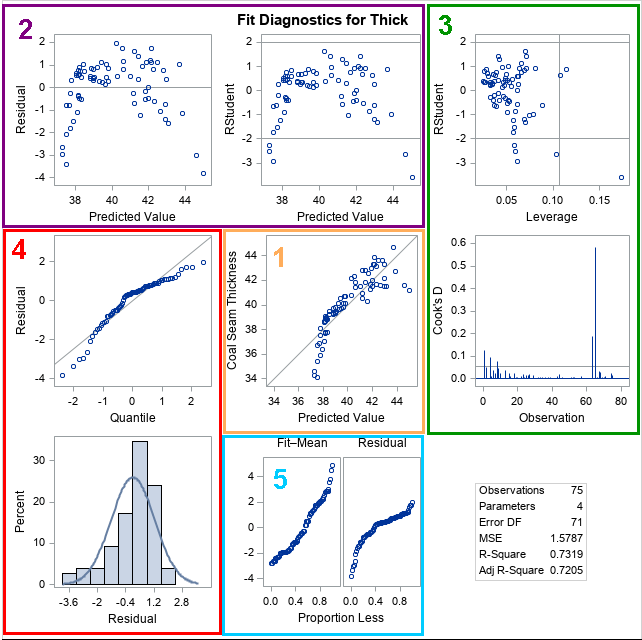The panel of diagnostic plots is shown. The panel of residual plots is shown later in this article. To guide the discussion, I have overlaid colored boxes around certain graphs. You can look at the graphs in any order, but I tend to look at them in the order indicated by the numbers in the panel.

### 1. The predicted versus observed response

The graph in the center (orange box) shows the quality of the predictive model. The graph plots the observed response versus the predicted response for each observation. For a model that fits the data well, the markers will be close to the diagonal line. Markers that are far from the line indicate observations for which the predicted response is far from the observed response. That is, the residual is large.

For this model, the cloud of markers is not close to the diagonal, which indicates that the model does not fit the data. You can see several markers that are far below the diagonal. These observations will have large negative residuals, as shown in the next section.

### 2. The residual and studentized residual plots

Two residual plots in the first row (purple box) show the raw residuals and the (externally) studentized residuals for the observations.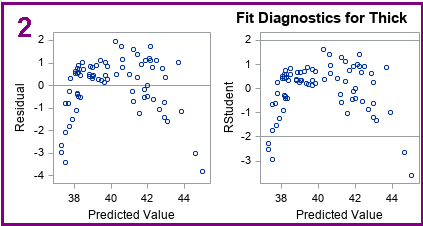The first graph is a plot of the raw residuals versus the predicted values. Ideally, the graph should not show any pattern. Rather, it should look like a set of independent and identically distributed random variables. You can use this graph for several purposes:

1. If the residuals seem to follow a curve (as in this example), it indicates that the model is not capturing all the "signal" in the response variable. You can try to add additional effects to the model to see if you can eliminate the pattern.
2. If the residuals are "fan shaped," it indicates that the residuals do not have constant variance. Fan-shaped residuals are an example of heteroscedasticity. The canonical example of a fan-shaped graph is when small (in magnitude) residuals are associated with observations that have small predicted values. Similarly, large residuals are associated with observations that have large predicted values. Heteroscedasticity does not invalidate the model's ability to make predictions, but it violates the assumptions behind inferential statistics such as p-values, confidence intervals, and significance tests.
3. Detect autocorrelation. If the residuals are not randomly scattered, it might indicate that they are not independent. A time series can exhibit autocorrelation; spatial data can exhibit spatial correlations.

The second residual graph often looks similar to the plot of the raw residuals. The vertical coordinates in the second graph (labeled "RStudent") are externally studentized residuals. The PROC REG documentation includes the definition of a studentized residual. For a studentized residual, the variance for the i_th observation is estimated without including the i_th observation. If the magnitude of the studentized residual exceeds 2, you should examine the observation as a possible outlier. Thus, the graph includes horizontal lines at ±2. For these data, five observations have large negative residuals.

### 3. Influential observations and high-leverage points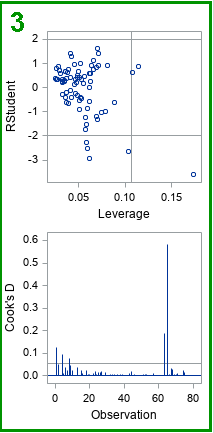The two graphs in the upper right box (green) enable you to investigate outliers, influential observations, and high-leverage points. One graph plots the studentized residuals versus the leverage value for each observation. As mentioned previously, the observations whose studentized residuals exceed ±2 can be considered outliers. The leverage statistic attempts to identify influential observations. The leverage statistic indicates how far an observation is from the centroid of the data in the space of the explanatory variables. Observations far from the centroid are potentially influential in fitting the regression model. "Influential" means that deleting that observation can result in a relatively large change in the parameter estimates.

Markers to the right of the vertical line are high-leverage points. Thus, the three lines in the graph separate the observations into four categories: (1) neither an outlier nor a high-leverage point; (2) an outlier, but not a high-leverage point; (3) a high-leverage point, but not an outlier; and (4) an outlier and a high-leverage point.

If there are high-leverage points in your data, you should be careful interpreting the model. Least squares models are not robust, so the parameter estimates are affected by high-leverage points.

The needle plot of Cook's D second graph (second row, last column) is another plot that indicates influential observations. Needles that are higher than the horizontal line are considered influential.

A subsequent article includes details about how to use the Cook's D plot to identify influential observations in your data.

### 4. Normality of residuals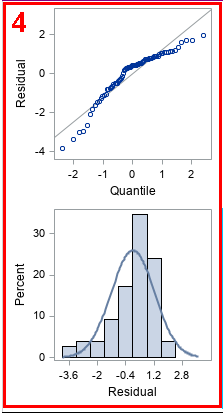The graphs in the lower left (red box) indicate whether the residuals for the model are normally distributed. Normally distributed residuals are one of the assumptions of regression that are used to derive inferential statistics. The first plot is a normal quantile-quantile plot (Q-Q plot) of the residuals. If the residuals are approximately normal, the markers should be close to the diagonal line. The following table gives some guidance about how to interpret deviations from a straight line:

Shape of Q-Q Plots
Point Pattern Interpretation
all but a few points fall on a line outliers in the data
left end of pattern is below the line long tail on the left
left end of pattern is above the line short tail on the left
right end of pattern is below the line short tail on the right
right end of pattern is above the line long tail on the right

For the Q-Q plot of these residuals, the markers are below the diagonal line at both ends of the pattern. That means that the distribution of residuals is negatively skewed: it has a long tail on the left and a short tail on the right. This is confirmed by looking at the histogram of residuals. For this model, the residuals are not normal; the overlay of the normal density curve does not fit the histogram well.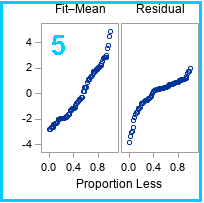The last graph (blue box) is the residual-fit spread plot, which I have covered in a separate article. For these data, you can conclude that the three explanatory variables account for a significant portion of the variation in the model.

### Residual plots with smoothers

Lastly, the PLOTS=RESIDUALPLOT(SMOOTH) option on the PROC REG statement creates a panel that includes the residuals plotted against the value of each explanatory variable. The SMOOTH suboption overlays a loess smoother, which is often helpful in determining whether the residuals contain a signal or are randomly distributed. The panel for these data and model is shown below: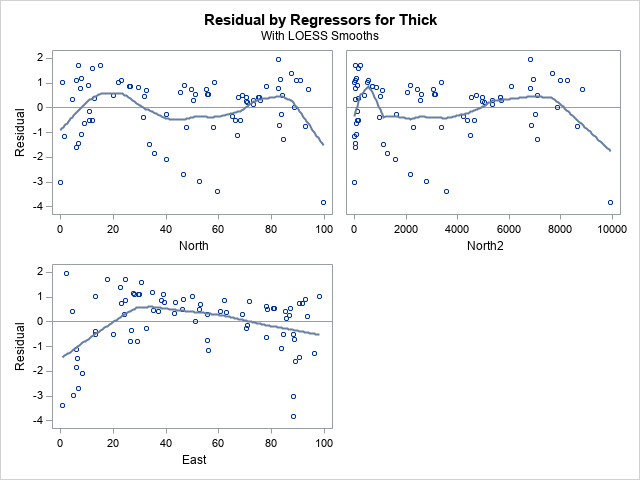The residuals plotted again the East variable shows a systematic pattern. It indicates that the model does not adequately capture the dependence of the response on the East variable. An analyst who looks at this plot might decide to add a quadratic effect in the East direction, or perhaps to model a fully quadratic response surface by including the North*East interaction term.

### Summary

This article created a regression model that does not fit the data. The regression diagnostic panel detects the shortcomings in the regression model. The diagnostic panel also shows you important information about the data, such as outliers and high-leverage points. The diagnostic plot can help you evaluate whether the data and model satisfy the assumptions of linear regression, including normality and independence of errors.

A subsequent article will describe how to use the diagnostic plots to identify influential observations.

The post An overview of regression diagnostic plots in SAS appeared first on The DO Loop.When you fit a regression model, it is useful to check diagnostic plots to assess the quality of the fit. SAS, like most statistical software, makes it easy to generate regression diagnostics plots. Most SAS regression procedures support the PLOTS= option, which you can use to generate a panel of diagnostic plots. Some procedures (most notably PROC REG and PROC LOGISTIC) support dozens of graphs that help you to evaluate the fit of the model, to determine whether the data satisfy various assumptions, and to identify outliers and high-leverage points. Diagnostic plots are most useful when the size of the data is not too large, such as less than 5,000 observations.

This article shows how to interpret diagnostic plots for a model that does not fit the data. For an ill-fitting model, the diagnostic plots should indicate the lack of fit.

This article discusses the eight plots in the DiagnosticsPanel plot, which is produced by several SAS regression procedures. To make the discussion as simple as possible, this article uses PROC REG to fit an ordinary least squares model to the data.

The eight plots can be classified into five groups:

1. A plot of the observed versus predicted responses. (Center of panel.)
2. Two graphs of residual values versus the predicted responses. (Upper left of panel.)
3. Two graphs that indicate outliers, high-leverage observations, and influential observations. (Upper right of panel.)
4. Two graphs that assess the normality of the residuals. (Lower left of panel.)
5. A plot that compares the cumulative distributions of the centered predicted values and the residuals. (Bottom of panel.)

This article also includes graphs of the residuals plotted against the explanatory variables.

### Create a model that does not fit the data

This section creates a regression model that (intentionally) does NOT fit the data. The data are 75 locations and measurements of the thickness of a coal seam. A naive model might attempt to fit the thickness of the coal seam as a linear function of the East-West position (the East variable) and the South-North position (the North variable). However, even a cursory glance at the data (see Figure 2 in the documentation for the VARIOGRAM procedure) indicates that the thickness is not linear in the North-South direction, so the following DATA step creates a quadratic effect. The subsequent call to PROC REG fits the model to the data and uses the PLOT= option to create a panel of diagnostic plots. By default, PROC REG creates a diagnostic panel and a panel of residual plots. If you want to add a loess smoother to the residual plots, you can use the SMOOTH suboption to the RESIDUALPLOT option, as follows:

data Thick2; set Sashelp.Thick; North2 = North**2; /* add quadratic effect */ run;   proc reg data=Thick2 plots =(DiagnosticsPanel ResidualPlot(smooth)); model Thick = North North2 East; quit;`The panel of diagnostic plots is shown. The panel of residual plots is shown later in this article. To guide the discussion, I have overlaid colored boxes around certain graphs. You can look at the graphs in any order, but I tend to look at them in the order indicated by the numbers in the panel.

### 1. The predicted versus observed response

The graph in the center (orange box) shows the quality of the predictive model. The graph plots the observed response versus the predicted response for each observation. For a model that fits the data well, the markers will be close to the diagonal line. Markers that are far from the line indicate observations for which the predicted response is far from the observed response. That is, the residual is large.

For this model, the cloud of markers is not close to the diagonal, which indicates that the model does not fit the data. You can see several markers that are far below the diagonal. These observations will have large negative residuals, as shown in the next section.

### 2. The residual and studentized residual plots

Two residual plots in the first row (purple box) show the raw residuals and the (externally) studentized residuals for the observations.The first graph is a plot of the raw residuals versus the predicted values. Ideally, the graph should not show any pattern. Rather, it should look like a set of independent and identically distributed random variables. You can use this graph for several purposes:

1. If the residuals seem to follow a curve (as in this example), it indicates that the model is not capturing all the "signal" in the response variable. You can try to add additional effects to the model to see if you can eliminate the pattern.
2. If the residuals are "fan shaped," it indicates that the residuals do not have constant variance. Fan-shaped residuals are an example of heteroscedasticity. The canonical example of a fan-shaped graph is when small (in magnitude) residuals are associated with observations that have small predicted values. Similarly, large residuals are associated with observations that have large predicted values. Heteroscedasticity does not invalidate the model's ability to make predictions, but it violates the assumptions behind inferential statistics such as p-values, confidence intervals, and significance tests.
3. Detect autocorrelation. If the residuals are not randomly scattered, it might indicate that they are not independent. A time series can exhibit autocorrelation; spatial data can exhibit spatial correlations.

The second residual graph often looks similar to the plot of the raw residuals. The vertical coordinates in the second graph (labeled "RStudent") are externally studentized residuals. The PROC REG documentation includes the definition of a studentized residual. For a studentized residual, the variance for the i_th observation is estimated without including the i_th observation. If the magnitude of the studentized residual exceeds 2, you should examine the observation as a possible outlier. Thus, the graph includes horizontal lines at ±2. For these data, five observations have large negative residuals.

### 3. Influential observations and high-leverage pointsThe two graphs in the upper right box (green) enable you to investigate outliers, influential observations, and high-leverage points. One graph plots the studentized residuals versus the leverage value for each observation. As mentioned previously, the observations whose studentized residuals exceed ±2 can be considered outliers. The leverage statistic attempts to identify influential observations. The leverage statistic indicates how far an observation is from the centroid of the data in the space of the explanatory variables. Observations far from the centroid are potentially influential in fitting the regression model. "Influential" means that deleting that observation can result in a relatively large change in the parameter estimates.

Markers to the right of the vertical line are high-leverage points. Thus, the three lines in the graph separate the observations into four categories: (1) neither an outlier nor a high-leverage point; (2) an outlier, but not a high-leverage point; (3) a high-leverage point, but not an outlier; and (4) an outlier and a high-leverage point.

If there are high-leverage points in your data, you should be careful interpreting the model. Least squares models are not robust, so the parameter estimates are affected by high-leverage points.

The needle plot of Cook's D second graph (second row, last column) is another plot that indicates influential observations. Needles that are higher than the horizontal line are considered influential.

A subsequent article includes details about how to use the Cook's D plot to identify influential observations in your data.

### 4. Normality of residualsThe graphs in the lower left (red box) indicate whether the residuals for the model are normally distributed. Normally distributed residuals are one of the assumptions of regression that are used to derive inferential statistics. The first plot is a normal quantile-quantile plot (Q-Q plot) of the residuals. If the residuals are approximately normal, the markers should be close to the diagonal line. The following table gives some guidance about how to interpret deviations from a straight line:

Shape of Q-Q Plots
Point Pattern Interpretation
all but a few points fall on a line outliers in the data
left end of pattern is below the line long tail on the left
left end of pattern is above the line short tail on the left
right end of pattern is below the line short tail on the right
right end of pattern is above the line long tail on the right

For the Q-Q plot of these residuals, the markers are below the diagonal line at both ends of the pattern. That means that the distribution of residuals is negatively skewed: it has a long tail on the left and a short tail on the right. This is confirmed by looking at the histogram of residuals. For this model, the residuals are not normal; the overlay of the normal density curve does not fit the histogram well.The last graph (blue box) is the residual-fit spread plot, which I have covered in a separate article. For these data, you can conclude that the three explanatory variables account for a significant portion of the variation in the model.

### Residual plots with smoothers

Lastly, the PLOTS=RESIDUALPLOT(SMOOTH) option on the PROC REG statement creates a panel that includes the residuals plotted against the value of each explanatory variable. The SMOOTH suboption overlays a loess smoother, which is often helpful in determining whether the residuals contain a signal or are randomly distributed. The panel for these data and model is shown below:The residuals plotted again the East variable shows a systematic pattern. It indicates that the model does not adequately capture the dependence of the response on the East variable. An analyst who looks at this plot might decide to add a quadratic effect in the East direction, or perhaps to model a fully quadratic response surface by including the North*East interaction term.

### Summary

This article created a regression model that does not fit the data. The regression diagnostic panel detects the shortcomings in the regression model. The diagnostic panel also shows you important information about the data, such as outliers and high-leverage points. The diagnostic plot can help you evaluate whether the data and model satisfy the assumptions of linear regression, including normality and independence of errors.

A subsequent article will describe how to use the diagnostic plots to identify influential observations.

The post An overview of regression diagnostic plots in SAS appeared first on The DO Loop.